图像处理算法评价_图像处理算法 和视频处理算法的区别 - CSDN
• 图像处理原理 关于图象，要明确图片是由各个相素点组合而成，每个相素点又是由R，G，B不同比例混和而成，当明白了成相原理，当我们想对图片进行颜色处理，譬如滤镜效果，就可以通过改变R，G，B的比例值从而达到...

图像处理原理

关于图象，要明确图片是由各个相素点组合而成，每个相素点又是由R，G，B不同比例混和而成，当明白了成相原理，当我们想对图片进行颜色处理，譬如滤镜效果，就可以通过改变R，G，B的比例值从而达到我们期望的颜色效果。

分析
安卓中提供图片颜色处理的方法类，可以进行色相，明度，饱和度的调整来实现对图片颜色特效的处理，在色彩丰富的彩屏上，颜色的渲染效果很好，图片处理结果也很好，而在电子书上，由于是墨水屏，可支持的色调就是16种可配的黑白灰，所以最好是将图片处理为灰度图片，再进行显示，效果会更加美观。进行图象处理，调配RGB的值，实质上也就是一些图象处理的配比算法，比如运用在电子书上的灰度算法就是RGB三者的配比相同。

算法代码：
public Bitmap ConvertGrayImg(int resID)
{
Bitmapimg1=((BitmapDrawable)getResources().getDrawable(resID)).getBitmap();  //首先要得到原图
//得到原图的长，宽，即图片的大小
int w=img1.getWidth(),h=img1.getHeight();
int[] pix = new int[w * h];  //设置一个和图片大小一样大的数组，用于存放相素值
img1.getPixels(pix, 0, w, 0, 0, w, h);  //得到相素点的值
int alpha=0xFF<<24;
for (int i = 0; i < h; i++) {
for (int j = 0; j < w; j++) {
// 获得像素的颜色
int color = pix[w * i + j];
int red = ((color & 0x00FF0000) >> 16);
int green = ((color & 0x0000FF00) >> 8);
int blue = color & 0x000000FF;
color = (red + green + blue)/3;
color = alpha | (color << 16) | (color << 8) | color;
pix[w * i + j] = color;  //将改变后的像素值重置
}
}
Bitmap result=Bitmap.createBitmap(w, h, Config.ARGB_4444);  //创建一张新图 Config.ARGB_4444：这个参数，是可支持16种色度值
result.setPixels(pix, 0, w, 0, 0,w, h); //将最终改变后的的相素值设置给新的图片
return result;
}

分析结果

这段代码是显示在电子书上效果最好的，能够淡出图片过渡的感觉，没有那么分明的一块，一块的显示感觉。
不足的地方就是此款效率低，由于是通过遍历并修改每个像素点的值，所以比较消耗时间，不过处理速度比较快，因为图片的大小都不大，处理出结果也是非常快的，其实一般做开发，采取的都是用安卓给我们提供的类方法进行图象的处理，速度快且效率高。然而安卓中处理成灰度效果的接口并不适用于电子书上，效果不好。

自定义算法和安卓内置API的性能对比显然，API的效率快，不过在电子书上的显示效果不理想。

最后总结

关于图片处理，循环遍历每个相素点，对于较小的图片，效果好且用时不是太多（小于100KB），如果图片大于300KB，用户体验就差了，所以可在图片小于100KB的时候进行图像算法处理，否则不予处理。

展开全文• 图像质量评价性能标准 SROCC KROCC PLCC rMSE
The ‘good-ness’ of any algorithm is gauged by measuring the correlation of algorithmic scores with subjective (differential) mean opinion scores (DMOS/MOS) on a large dataset spanning different distortion.

正如以上所说对于一种图像质量评价算法性能好坏的评估就是看在具有不同失真的大数据集上观察者的主观评分和算法评分的相关度。如果它们的相关度较高，则说明该质量评价算法的性能较好，否则反之。

那么什么是相关呢？简单的说就是当一个或几个相互联系的变量取一定数值时，与之相对应的另一个变量的值虽然不确定，但它仍然按某种规律在一定范围内变化，变量间的这种关系，被称为相关关系。相关关系可以用相关系数来评价，其绝对值越高表明其越相关，否则反之。相关系数的取值一般在-1到1之间。可以回想一下我们学过的概率论与数理统计中的相关系数，当两个随机变量的相关系数的绝对值为1时，可以将一个随机变量表示为另一个随机变量的一元线性函数，这时变量的相关度最高。例如Y=a*X+b。但是当其相关系数的绝对值小于1时，就不会有这么明确的函数关系。此时的相关度较小。下图是两个变量相关的示例，图中的每一点是变量X，Y的一对取值。可以看出这两个变量近似于线性相关，其相关系数接近于1。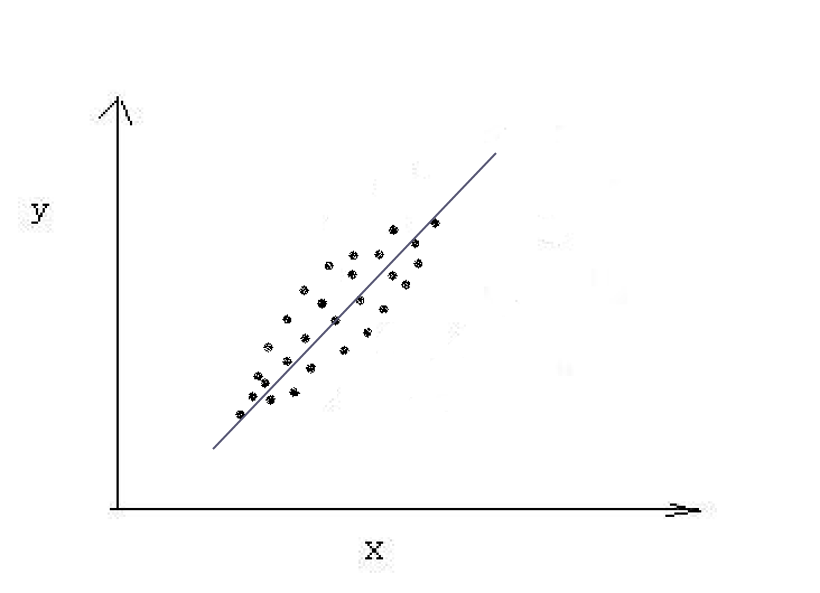现在用到的评价参数主要有四个。它们分别是SROCC,KROCC,PLCC和rMSE。下面分别对它们进行具体介绍。因为现在由于做图像质量评价的需要，我只是大概了解一下它们所表达的含义以及它们的计算方法，可能不像统计学中那么的专业。

1.  SROCC

SROCC的全称是the Spearman rank-order correlation coefficient，又简称为Spearman’s rho。下面我先例举一个具体的例子，然后对其进行具体介绍。

假设现在有10个学生的数学(X)和英语(Y)成绩，现在我们要来判断X和Y的相关性。

num
X
Y
Rank(X)
Rank(Y)
d
d2$d^2$

1
56
66
9
4
5
25

2
75
70
3
2
1
1

3
45
40
10
10
0
0

4
71
60
4
7
-3
9

5
62
65
6
5
1
1

6
64
56
5
9
-4
16

7
58
59
8
8
0
0

8
80
77
1
1
0
0

9
76
67
2
3
-1
1

10
61
63
7
6
1
1

如上表所示，X列表示某个学生的数学成绩，Y列表示其对应的英语成绩。然后分别对数学成绩和英语成绩进行等级划分，分数最高的划分为1，最低的划分为10，其它的以此分别划分为2到9之间的一个整数。划分后如Rank(X)列和Rank(Y)列所示。然后对得到的每一个同学的数学成绩等级减去英语成绩等级得到数据列d，然后对d平方得到d的平方数据列。在这个过程中如果某几个同学的某一门成绩相同，例如张三和李四的数学成绩都是70，假设它们被划分的等级分别为3和4，反之亦然，则需要先对等级划分进行平均，这里等级平均后是3.5，然后把它们的等级划分都定为3.5。下面我们就可以来计算它们的SROCC了。

公式一：
srocc=1−6∑ni=1d2in(n2−1)srocc=1-\frac{6\sum_{i=1}^n d_i^2}{n\left(n^2-1\right)}

公式二：
srocc=∑ni=1(ui−u¯)(vi−v¯)∑ni=1(ui−u¯)2∑ni=1(vi−v¯)−−−−−−−−−−−−−−−−−−−−−−√srocc=\frac{\sum_{i=1}^n (u_i-\bar u)(v_i-\bar v)}{\sqrt {\sum_{i=1}^n (u_i- \bar u)^2 \sum_{i=1}^n(v_i- \bar v)}}

对于以上两个公式，当某个特定的数据集合(如数学成绩)中有相等的值时使用公式二，否则使用公式一。其中n为采集的数据样本中数据对的个数，上例中n为10。其中u¯$\bar u$和v¯$\bar v$分别为某个特定的数据集合在完成等级划分之后的等级平均值，比如上例中数学成绩的等级平均。ui$u_i$和vi$v_i$分别对相应数据对的等级划分。

我们从以上例子可以看出srocc主要评价的是两组数据的等级相关性。也就是对于两个变量的一些数据对(如上例所示)，数据对的意思是当一个变量取一个值时观察到另一个变量取到的值，当对每个变量的数据进行等级划分后，我们可以得到每个变量的等级序列，如上例中的Rank(X)和Rank(Y),若两个变量的srocc越高则表明它们的等级相关性较高(比如对于上例中任意的i，若Rank(xi)$Rank(x_i)$=Rank(yi)$Rank(y_i)$,则表明它们的等级相关非常高，srocc=1)，否则反之。

2.KROCC

KROCC的全称是the Kendall rank-order correlation  coefficient，又简称为Kendall’s tau。和srocc一样这里我也是通过例子计算来解释它。在这里我还是用srocc中的那个例子。

在这里我先讲一些基本的概念。假设我们现在需要评价两个变量X和Y的相关性，(x1,y1)$(x_1,y_1)$ ,(x2,y2)$(x_2,y_2)$,……., (xn,yn)$(x_n,y_n)$是变量X和Y的一些数据对，(xi,yi)$(x_i,y_i)$可以看做是上面例子中第i个学生的数学成绩和英语成绩组成的数据对。现在我们从这n个数据对中任意选两个数据对(xi,yi)$(x_i,y_i)$和(xj,yj)$(x_j,y_j)$形成[(xi,yi),(xj,yj)]$[(x_i,y_i),(x_j,y_j)]$，其中i!=j且[(xi,yi),(xj,yj)]$[(x_i,y_i),(x_j,y_j)]$和[(xj,yj),(xi,yi)]$[(x_j,y_j),(x_i,y_i)]$，这样的对子一种有N=n(n+1)2$N=\frac{n(n+1)} 2$种。对于任意的[(xi,yi),(xj,yj)]$[(x_i,y_i),(x_j,y_j)]$，若xi>xj$x_i>x_j$且yi>yj$y_i>y_j$或者xi<xj$x_i且yi<yj$y_i则称呼这样的对子是concordant，它们的个数记为P。xi>xj$x_i>x_j$且yi<yj$y_i或者xi<xj$x_i且yi>yj$y_i>y_j$则称呼这样的对子discordant，它们的个数记为Q。若xi=xj$x_i=x_j$且yi>yj$y_i>y_j$或者xi=xj$x_i=x_j$且yi<yj$y_i，这样的对子个数记为X0$X_0$。若xi>xj$x_i>x_j$且yi=yj$y_i=y_j$或者xi<xj$x_i且yi=yj$y_i=y_j$，这样的对子个数记为Y0$Y_0$。若xi=xj$x_i=x_j$且yi=yj$y_i=y_j$，这样的对子的个数记为(XY)0$(XY)_0$。因此N=P+Q+X0$X_0$+Y0$Y_0$+(XY)0$(XY)_0$。

有了这些概念之后我们就可以来计算krocc了，下面就是其公式。

krocc=P−Q(P+Q+X0)(P+Q+Y0)−−−−−−−−−−−−−−−−−−−−−√krocc=\frac{P-Q} {\sqrt{(P+Q+X_0)(P+Q+Y_0)}}

从这里的概念的解释我们可以了解到，krocc也是评价两个变量的等级相关的，这里就不再累述了。

3.PLCC

PLCC的全称是Pearson product-moment correlation coefficient。因为它相对比较简单，所以我这里直接给出它的公式：
plcc=COV(X,Y)δXδYplcc=\frac{COV(X,Y)} {\delta_X\delta_Y}

对概率论和数理统计还比较熟悉的同学可以知道这就是我们学过的线性相关系数，若我们把X和Y看做两个随机变量，则分母是随机变量X和Y的协方差，分子是它们的标准差的乘积。plcc就是来评价两组数据的线性相关关系的。若其绝对值越接近于1，则其之间的关系就越可以用一个线性直线方程来表示。多余的我就不再累述了。

4.rMSE

rMSE的全称是root mean square error。其实他就是MSE(均方误差)的平方根。如果拿我举得的那个例子来说的话，那它的计算公式是：
rMSE=∑ni=1(xi−yi)2n−−−−−−−−−−−−−√rMSE=\sqrt\frac{\sum_{i=1}^n{(x_i-y_i)^2}}{n}
其中(xi,yi)$(x_i,y_i)$是对应的数学成绩和英语成绩的数据对。直面理解rMSE就是数据错误平方均值的平方根。这里只是一种离散情况的简单解释，更复杂的情况读者可以参阅我参考资料中的解释和其它方面的资料。当然其值应该越小越好，这样它的错误就小啊！而上面的三个系数对于一种质量评价算法来说应该越大越好，这样的话预测值就和主观评价值越接近，这样的质量评价算法肯定好啊！

最后再说几句，srocc，krocc和plcc在matlab中可以直接利用函数corr计算。但是在计算plcc和rMSE的时候需要先将要比较的两组数据或者两个变量的数据(这个我也只是大概了解一下，想详细了解的同学要自己查资料了)进行一下非线性回归分析或拟合之类的操作然后再去算。matlab中可以用nlinfit这个函数。
参考资料：
1:https://statistics.laerd.com/statistical-guides/spearmans-rank-order-correlation-statistical-guide.php

2:http://www.epixanalytics.com/modelassist/AtRisk/Model_Assist.htm#Probability_theory_and_statistics/The_basics/Probability_theorems/Useful_concepts/Rank_Order_Correlation_Coefficient.htm

3:http://blog.csdn.net/wsywl/article/details/5859751

4:https://en.wikipedia.org/wiki/Spearman%27s_rank_correlation_coefficient

5:http://blog.csdn.net/wsywl/article/details/5889419

6:https://onlinecourses.science.psu.edu/stat509/node/158

7:https://en.wikipedia.org/wiki/Kendall_rank_correlation_coefficient

8:http://blog.csdn.net/wsywl/article/details/5727327

9:https://en.wikipedia.org/wiki/Pearson_product-moment_correlation_coefficient

10:https://en.wikipedia.org/wiki/Mean_squared_error

11:https://en.wikipedia.org/wiki/Root-mean-square_deviation
展开全文图像处理 标准 性能
• ## 图像评价指标

万次阅读 2017-06-25 17:24:30
1.图像评价指标　图像评价指标用来客观评价图像处理算法的优劣性，一般在对比实验中突出自己提出的算法的亮点。一般来说，每个细分的领域都有相应的指标，如边缘检测，有PFOM（Pratt’s Figure Of Merit）...
1.图像评价指标

图像评价指标用来客观评价图像处理中算法的优劣性，一般在对比实验中突出自己提出的算法的亮点。一般来说，每个细分的领域都有相应的指标，如边缘检测，有PFOM（Pratt’s Figure Of Merit）（第一个引用是它的来源，第二个引用是使用它的例子）；图像增强，有EEME（Evaluating image Enhancement Measure by Entropy）。
还有一个比较大的领域–图像复原，包括图像去噪、去模糊、去雾等的评价指标基本上是通用的，都可以用复原后图像与参考图像对比来进行评价，即有参考图像评价指标。相应地提出一系列的指标：(R)MSE, (M)SSIM, PSNR(CSNR)等等，这些指标比较常见。原则上来说，采用越通用的指标，如去噪大家都采用PSNR和SSIM，这样得到的结果也越令人信服。#每种指标都有具体针对的情况，根据评价的目的和情况来设计评价算法。
接下来介绍几种评价指标：

2.SMD2

图像的清晰度可以这样得到：对于模糊的图像，其整个图像的灰度较为均匀，相邻像素之间的差值会很小，相乘之后的结果会很小；图像越清晰，那么，边缘跟其周围像素灰度的差值也越大，相乘之后的值也会更大，这样就可以定义一个指标：灰度方差乘积（SMD2），它可以快速准确地来判断一副图像清晰的程度。 SMD2=∑∑|I(x,y)−I(x+1,y)|∗|I(x,y)−I(x,y+1)|<!--//--><![CDATA[//><!--
SMD2=\sum\sum|I(x,y)-I(x+1,y)|*|I(x,y)-I(x,y+1)|
//--><!]]>
用代码实现如下：

double getSMD2(const Mat& test)//SMD2=sum(|test(x,y)-test(x+1,y)|*|test(x,y)-test(x,y+1)|)
{
double temp = 0;
const uchar* ix;
const uchar* ix1;
for (int i = 0; i < test.rows-1; ++i)
{
ix = test.ptr<uchar>(i);
ix1 = test.ptr<uchar>(i + 1);
for (int j = 0; j < test.cols-1; ++j)
{
temp += abs(ix[j] - ix1[j])*abs(ix[j] - ix[j + 1]);
}
}
return temp / (test.rows * test.cols);
}


3. 人眼特性评价指标HVSNR

人类的视觉感知有3个显著的特性，即视觉非线性特性（Weber定律）、视觉敏感度带通和视觉多通道及掩盖效应。利用小波变换与HVS多通道特性相匹配的特点，建立一种和人的视觉评价保持良好一致的方法，其评价结果与主观评价平均评价分数的相关系数达0.95，而对应的客观评价方法与主观评价平均评价分数的相关系数为0.81。利用对比度敏感度函数的带通特性以简化HVS模型，将不同空间频带失真用Minkowski求和进行非线性合并，最后仿照峰值信噪比的定义，得到结果。具体的步骤是：
(1)将待测图像和标准图像选用D9/7小波进行分解，分解为4级；
(2)将4级小波分为5个频带，对不同空间频带乘于对应的CSF系数；
(3)待测图像和标准图像相减得到5个频带的误差E，然后按照下面公式进行求和： S=(∑n=1N|En|β)1/β<!--//--><![CDATA[//><!--
S=(\sum_{n=1}^{N}|E_n|^\beta)^{1/\beta}
//--><!]]>
(4)根据信噪比的定义得到基于人眼视觉特性的信噪比结果： HVSRN=10log[(2552)/s]<!--//--><![CDATA[//><!--
HVSRN=10log[(255^2)/s]
//--><!]]>double **fwt97(double** matrix, int width, int height)
{
//9 / 7 Coefficients:
double a1 = -1.586134342;
double a2 = -0.05298011854;
double a3 = 0.8829110762;
double a4 = 0.4435068522;

//Scale coeff:
double k1 = 0.81289306611596146; // 1 / 1.230174104914
double k2 = 0.61508705245700002; // 1.230174104914 / 2

for (int col = 0; col < width; ++col)
{
//Predict 1. y1
for (int row = 1; row < height - 1; row += 2)//奇数列
{
matrix[row][col] += a1 * (matrix[row - 1][col] + matrix[row + 1][col]);
}
matrix[height - 1][col] += 2 * a1 * matrix[height - 2][col];

//Update 1. y0
for (int row = 2; row < height; row += 2)//偶数列
{
matrix[row][col] += a2 * (matrix[row - 1][col] + matrix[row + 1][col]);//这里注意不要越界
}
matrix[col] += 2 * a2 * matrix[col];

//Predict 2.
for (int row = 1; row < height - 1; row += 2)//奇数列
{
matrix[row][col] += a3 * (matrix[row - 1][col] + matrix[row + 1][col]);
}
matrix[height - 1][col] += 2 * a3 * matrix[height - 2][col];

//Updata 2.
for (int row = 2; row < height; row += 2)//偶数列
{
matrix[row][col] += a4 * (matrix[row - 1][col] + matrix[row + 1][col]);//
}
matrix[col] += 2 * a4 * matrix[col];
}

double **temp;
createMatrix(temp, Size(width, height));
for (int row = 0; row < height; ++row)
{
for (int col = 0; col < width; ++col)
{
if (row % 2 == 0)
temp[col][row / 2] = k1 * matrix[row][col];
else
temp[col][row / 2 + height / 2] = k2 * matrix[row][col];
}
}
for (int row = 0; row < height; ++row)
{
for (int col = 0; col < width; ++col)
{
matrix[row][col] = temp[row][col];
}
}

releaseMatrix(temp, Size(width, height));

return matrix;
}

Mat fwt97_2d(Mat image, int nlevels)
{
int iWidth = image.rows, iHeight = image.cols;
double **matrix;
createMatrix(matrix, image.size());

//convert mat to 2d matrix
const uchar *ix;
for (int row = 0; row < iHeight; ++row)
{
ix = image.ptr<uchar>(row);
for (int col = 0; col < iWidth; ++col)
{
matrix[row][col] = double(uchar(ix[col]));
}
}

int width = iWidth, height = iHeight;
//do the wavelet decompose
for (int i = 0; i < nlevels; ++i)
{
matrix = fwt97(matrix, width, height);
matrix = fwt97(matrix, width, height);
width /= 2;
height /= 2;
}

#ifdef SHOW_WAVELET
Mat im1(image.size(), CV_8UC1);
for (int row = 0; row < iHeight; ++row)
{
for (int col = 0; col < iWidth; ++col)
{
if (matrix[row][col] < 0)
im1.at<uchar>(row, col) = 0;
else if (matrix[row][col] > 255)
im1.at<uchar>(row, col) = 255;
else
im1.at<uchar>(row, col) = uchar(matrix[row][col]);
}
}
imshow("97wavelet", im1);
#endif
//multiple the CSF coefficient with different frequence band
double csf = { 2.16, 2.87, 3.16, 2.56 };
for (int i = 0; i < nlevels; ++i)
{
int tHeight = 0, tWidth = 0;
for (int row = tHeight; row < height; ++row)
{
for (int col = tWidth ; col < width; ++col)
{
matrix[row][col] = csf[i] * matrix[row][col];
}
}
tWidth = width;
tHeight = height;
width *= 2;
height *= 2;
}

Mat im(image.size(), CV_64FC1);
for (int row = 0; row < iHeight; ++row)
{
double * dm = im.ptr<double>(row);
for (int col = 0; col < iWidth; ++col)
{
dm[col] = matrix[row][col];
}
}
releaseMatrix(matrix, image.size());
return im;
}

double getHVSNR(const Mat &test, const Mat &reference, int nlevels)//the image size must be 2^n*2^n
{
Mat _test(test.size(), CV_64FC1), _ref(reference.size(), CV_64FC1);
_test = fwt97_2d(test, nlevels);
_ref = fwt97_2d(reference, nlevels);

//Minkovski nonlinear summation
Mat diff(test.size(), CV_64FC1), powImg(test.size(), CV_64FC1);
absdiff(_test, _ref, diff);
pow(diff, 4, powImg);

double temp = 0;
temp = mean(diff).val;
temp = pow(temp, 1. / 4);

return 10 * log10(255*255 / (temp+0.000001)); //add a small number avoiding the divider to be zero
}


4.PFOM

PFOM是由三种因子的组合来定义的：真实边缘的漏检测，伪边缘的误检测及边缘的定位误差，三种因子按下式组合起来就是Pratt品质因素： PFOM=1/max(Ne,Nd)∑Ndk=11/(1+βd(k)2)<!--//--><![CDATA[//><!--
PFOM=1/max(N_e,N_d )  ∑_{k=1}^{N_d}1/(1+βd(k)^2 )
//--><!]]>
其中，Ne<!--//--><![CDATA[//><!--
N_e
//--><!]]>是设定为参考边缘点的数目，Nd<!--//--><![CDATA[//><!--
N_d
//--><!]]>是算法提取到的边缘点的数目，β设为常数1/9，d(k)是第k个真实边缘点到检测到边缘点之间的欧式距离： d(k)=||Ne−Nd||Eucild<!--//--><![CDATA[//><!--
d(k)=||N_e-N_d ||_{Eucild}
//--><!]]>
Pratt品质因素是一个范围从0到1的保真函数，这里的真实边缘Ne<!--//--><![CDATA[//><!--
N_e
//--><!]]>是有监督的人为数据，即为参考边缘。通常的做法有以Canny检测的结果作为参考边缘来计算，还有一种是，采用BSD dataset中的ground truth来作为true edge map。

function [F, fac, msc] = pratt(Ea,Ed)

% Function EDPM : Edge detector performance measure function.
%             Calculates for a given edge image the false alarm
%         count, miss count and figure of merit (F) values.
%
%
%    Input(s)... Ea : Actual edge image
%        Ed : Detected edge image.
%
%    Output(s).. fac: False alarm count
%        msc: miss count
%        F  : Figure of merit
Ea=double(Ea);
Ed=double(Ed);

[N,M]=size(Ea);
if [N,M]~=size(Ed)
error('Actual and detected edge image sizes must be same');
end;
a=0.1; % edge shift penalty constant;
fac=length(find((Ea-Ed)==-1)); % False Alarm Count
msc=length(find((Ea-Ed)==1));  % Miss Count
Na=sum(sum(Ea));Nd=sum(sum(Ed));
c=1/max(Na,Nd);
[ia,ja]=find(Ea==1);
for l=1:Na
Aes(l)=Ed(ia(l),ja(l));
end;
mi=ia(find(Aes==0));
mj=ja(find(Aes==0));
F=c*sum(Aes);
for k=1:length(mi)
n1=0;n2=0;m1=0;m2=0;
while sum(sum(Ed(mi(k)-n1:mi(k)+n2,mj(k)-m1:mj(k)+m2)))<1
if mi(k)-n1>1 n1=n1+1;end;
if mi(k)+n2<N n2=n2+1;end;
if mj(k)-m1>1 m1=m1+1;end;
if mj(k)+m2<M m2=m2+1;end;
end;
di=max([n1 n2 m1 m2]);
F=F+c/(1+a*di^2);
end;

F = F*100;

5.EEME

EEME借用了 Michelson对比度公式：
Modulation=(Lmax−Lmin)/(Lmax+Lmin)<!--//--><![CDATA[//><!--
{\rm Modulation}=({\rm L}_{\max}-{\rm L}_{\min})/({\rm L}_{\max}+{\rm L}_{\min})
//--><!]]>
增大Lmin<!--//--><![CDATA[//><!--
L_{min}
//--><!]]>就能减小对比度 EME==maxϕ∈{ϕ}(EME(ϕ))maxϕ∈{ϕ}⎛⎝1k1k2∑l=1k2∑k=1k120lnIWmax;k,l−IWmin;k,lIWmax;k,l+IWmin;k,l+c⎞⎠<!--//--><![CDATA[//><!--
\eqalignno{{\rm EME}\!=&\,\max_{\phi\in\{\phi\}}\left({\rm EME}(\phi)\right)\cr=&\,\max_{\phi\in\{\phi\}}\!\left({1\over k_{1}k_{2}}\sum_{l=1}^{k_{2}}\sum_{k=1}^{k_{1}}20\ln{I_{\max;k,l}^{W}-I_{\min;k,l}^{W}\over I_{\max;k,l}^{W}+I_{\min;k,l}^{W}+c}\right)\cr&&}
//--><!]]>
因此，对比度越高，ＥＭＥ相应地越大，图像增强的效果也越明显。

 Pratt W K. Digital Image Processing[M]. Wiley-Interscience, 1978.
 Sheng Y, Dementrio L, EasleyR, Hamid K. A Shearlet Approach to Edge Analysis and Detection[J], IEEE Transactions on Image Processing. 2009, 18(5):929-941.
 S.S. Agaian, B. Silver, K.A. Panetta, Transform coefficient histogram-based image enhancement algorithms using contrast entropy, IEEE Trans. Image Process. 16 (3) (2007) 741–758.
 A. Ghani, N. Isa. Enhancement of low quality underwater image through integrated global and local contrast correction. Elsevier Applied Soft Computing. 37(2015) 332-344.
 李郁峰, 陈念年, 张佳成.  一种快速高灵敏度聚焦评价函数.  计算机应用研究, 2010, 4.
 丁绪星, 朱日宏, 李建欣.  一种基于人眼视觉特性的图像质量评价.  中国图象图形学报, 2004, 2.
 Michelson A. Studies in optics[M]. University of Chicago Press, 1927.

展开全文图像处理
• 对于一种图像处理方法，怎么样来判断该算法效果的好坏呢？除了人眼本身的观察，还可以用某种指标来量化评判，本文将总结一下图像质量评判的方法及实现。 图像质量评价分类（IQA:image quality assessment）： ...
前言：
对于一种图像处理方法，怎么样来判断该算法效果的好坏呢？除了人眼本身的观察，还可以用某种指标来量化评判，本文将总结一下图像质量评判的方法及实现。
图像质量评价分类（IQA:image quality assessment）：
图像质量评价目前来看主要分为两类：
（1）主观评价。即用人眼观察和评判图像，主观评价和人的感觉相一致，但是容易受到环境、心境等影响，而且大量评价图像时也显得不现实。
（2）客观评价。即采用算法进行评价，相比于主观评价，客观评价具有操作简单、成本低、易于解析和实现等优点，是图像质量评价的研究重点。所以本篇重点总结一下客观评价的几种方法。
客观评价算法根据其对参考图像的依赖程度， 可分成三类：( 1) 全参考: 和参考图像的所有像素点做对应比较; ( 2) 半参考: 只需要和参考图像上的部分统计特征做比较;( 3) 无参考: 不需要具体的参考图像。其中全参考算法是研究时间最长、发展最成熟的。
一、全参考评价：
全参考算法根据算法采用的技术路线， 可分为基于误差统计量的算法和基于 HVS 模型的算法。
1.基于误差统计量的算法的思路： 通过设计特征来比较失真图像和参考图像的局部差异, 然后在整幅图像上求出一个总的平均统计量, 并把这个统计量与图像质量关联起来。最简单的质量评价算法就是均方差(Mean Squared Error, MSE)和峰值信噪比(Peak Signal- Noise Ratio, PSNR)。MSE 和 PSNR 计算复杂度小，易于实现，在图像处理领域中广泛应用。但缺点是它们给出的数值与图像的感知质量之间没有必然联系。
2.基于 HVS 模型的算法的思路：通过对 HVS 的某些底层特性进行建模，将失真图像和参考图像之间的绝对误差映射为能被人眼觉察的 JND( Just noticeable difference) 单位。根据对 HVS 模型描述的侧重点不同，又可以将图像质量评价模型归结为基于误差灵敏度评价算法和基于结构相似度评价算法两类。
2.1基于误差灵敏度的质量评价算法
该方法可用图1描述，算法不同之处在于侧重点和处理方式上的区别。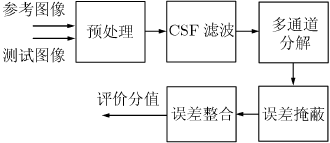图1.基于误差灵敏度的评价框架
该方法的缺点在于：
(a)基于 HVS 特征的方法一般认为原始图像质量是完美的，Weber 定律和点扩散函数(Point Spread Function)模型始终成立。
(b)一般假定 HVS 的多通道响应可以通过线性离散集合来模拟。
(c)一般假定通道分解是无损或无损于视觉的，变换后仍保持了质量评价的绝大部分信息。
(d)一般认为通道分解剔除了图像间的关联，道变换的作用可以通过掩蔽模型来模拟。
(e)HVS 的评价值可以通过测试误差的非线性组合来模拟，目前大多采用线性加权组合。

前 3 条假设从 HVS 的特点以及实际操作来说，相对比较合理。实验表明自然图像经过通道分解后，相同位置上的特征基本上相同或者相似，即各通道之间实际上存在较高的相关性，这与上述(d)点假设相矛盾。另外，误差的统计量来表征图像质量方法，论其如何加权组合，仍可能存在两幅图像失真类型完全不一样、但误差相同的现象。故上述(e)点假设也不合适。

2.2基于结构相似度的图像质量评价算法
自然图像具有特定的结构，素间有很强的从属关系，这些从属关系反映了视觉场景中的结构信息。由此产生了基于结构失真的图像质量评价方法，称为结构相似 (SSIM)方法，框架参见图2。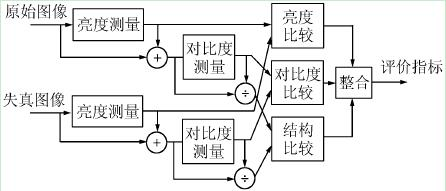图2.结构相似度评价框架
小结：基于误差灵敏度的方法通过人为模拟 HVS 对误差敏感度进行量化，过程易于解析，但是其算法过于繁杂；SSIM 评价方法通过测量图像结构信息的改变来反映图像质量的失真情况，算法上明显简化，但同时也屏蔽掉了 HVS的其它生理特征，过程不易于解析。所以，将 SSIM 和基于误差灵敏度的评价方法中采用的 HVS 特征加权评价联合起来，是图像质量评价今后的一个发展方向。

二、半参考评价：

半参考算法可以分为两种: 基于图像特征统计量的算法和基于数字水印的算法。这类算法的特点是其只需从参考图像中提取部分统计量用于比较, 无需原始的像素级别的信息。

三、无参考评价：
无参考算法可以分为两种: 针对失真类型的算法和基于机器学习的算法。这类方法的特点无需参考图像, 灵活性强。无参考算法的难点在于如何使评价结果尽量不受图像内容的影响。
衡量算法性能的定量指标：
图像质量评价算法应该具备以下特性:
( 1) 准确性：主观与客观评价值之间的差异较小;
( 2) 单调性: 客观评价值应随主观评价值的增减而增减;
( 3) 一致性: 算法在测试集上表现出的性能与其在训练集上表现的性能相近似。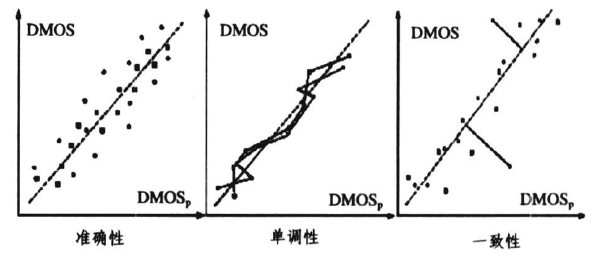图像质量评价算法实现：
这里附上几种图像质量评价指标的算法实现。
（1）峰值信噪比-PSNR（Peak Signal to Noise Ratio）
峰值信噪比（PSNR）经常用作图像压缩等领域信号重建质量的评价，常简单的方式是通过均方差（MSE）来定义：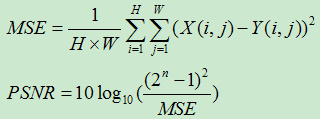MSE为当前图像 X 和参考图像 Y 的均方误差（Mean Square Error）。H、W 分别表示图像的高和宽；n为每像素的比特数，一般取8，即像素灰阶数为256。PSNR的单位是dB，数值越大表示失真越小。
    function pnsr_result = psnr(img_ref,img_in)
%   img_ref is a high reference quality image
%   img_in is the denoise image
%   pnsr_result is the PSNR of the denoise image
width = size(img_ref,2);
heigh = size(img_ref,1);
if( width ~= size(img_in,2) || heigh ~= size(img_in,1) )
disp('Please check whether the input image and reference image have same size');
return
end
[a,b]=size(img_ref);
XX=double(img_ref) - double(img_in);
mse_value = sum(sum( XX.^2 ))/(a*b);
pnsr_result = 10*log10( 255*255 / mse_value );
end
（2）SSIM
两张图像 X 和 Y 的结构相似性可按照以下方式求出: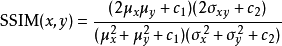,
其中 μ 是平均值，是的方差，σ是的方差，σxy是协方差。，是用来维持稳定的常数。L 是像素值的动态范围,k1=0.01,k2=0.03。结构相似性的范围为-1到1,当两张图像一模一样时，SSIM的值等于1。
结构相似度指数从图像组成的角度将结构信息定义为独立于亮度、对比度，反映场景中物体结构的属性，并将失真建模为亮度、对比度和结构三个不同因素的组合。均值作为亮度的估计，标准差作为对比度的估计，协方差作为结构相似程度的度量。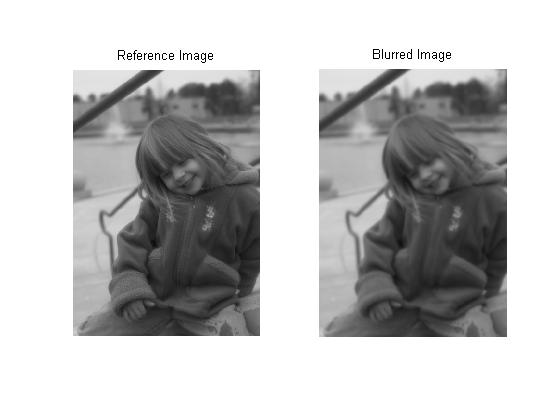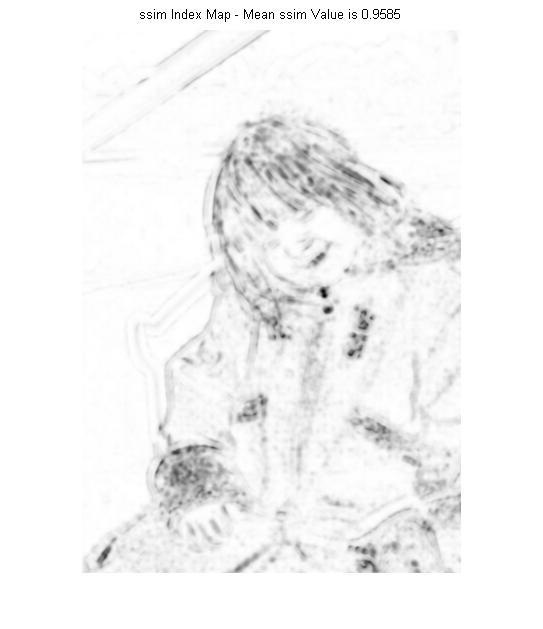（3）信噪比（SNR）
顾名思义，信噪比就是有用信号与噪声信号的比值，具体我直接附维基里的定义：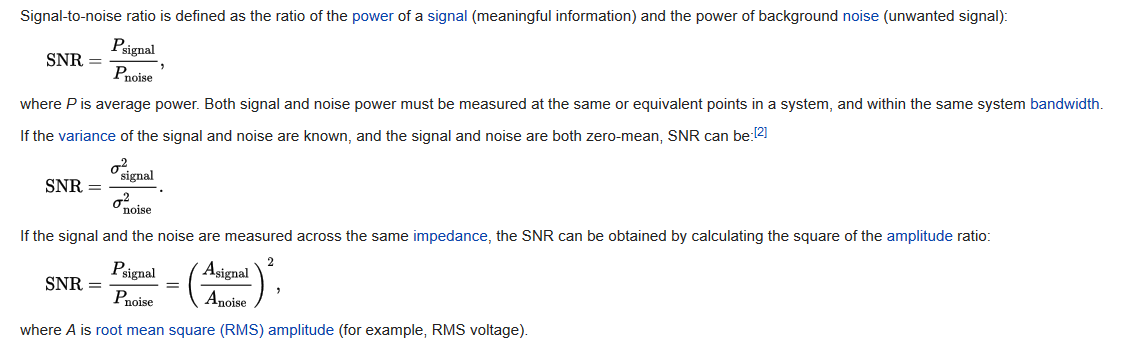matlab实现：
function snr=SNR2(I,In)
% 计算噪声比
% I :original signal
% In:noisy signal
% snr=10*log10(sigma2(I2)/sigma2(I2-I1))

[~,~,nchannel]=size(I);
snr=0;
I=double(I);
In=double(In);
if nchannel==1
Ps=sum(sum((I-mean(mean(I))).^2));%signal power
Pn=sum(sum((I-In).^2));%noise power
snr=10*log10(Ps/Pn);
elseif nchannel==3
for i=1:3
Ps=sum(sum((I(:,:,i)-mean(mean(I(:,:,i)))).^2));%signal power
Pn=sum(sum((I(:,:,i)-In(:,:,i)).^2));%noise power
snr=snr+10*log10(Ps/Pn);
end
snr=snr/3;
end测试函数结果：
%% snr
clc,clear all,close all;
H = fspecial('Gaussian',[11 11],1.5);
A = imfilter(ref,H,'replicate');
subplot(1,2,1); imshow(ref); title('Reference Image');
subplot(1,2,2); imshow(A);   title('Blurred Image');
snrValue=SNR2(ref,A )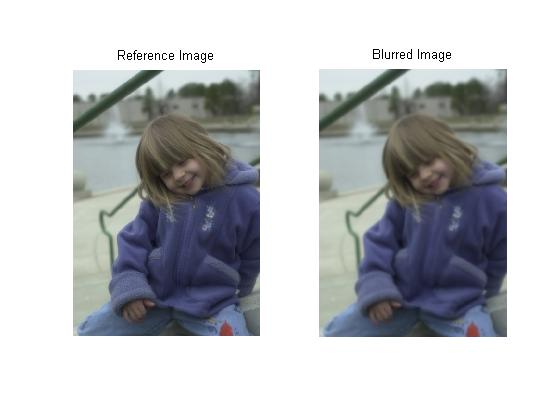snrValue =

25.6430
参考：
《图像质量评价研究综述》[J]. 计算机科学《图像质量研究方法进展》[J]. 电子与信息学报《基于频域的结构相似度的图像质量评价方法》[J]. 清华大学学报http://blog.csdn.net/purgle/article/details/73719101http://blog.csdn.net/ebowtang/article/details/43643037http://blog.csdn.net/xiaohaijiejie/article/details/48053595
展开全文• 嵌入式图像处理算法优化指南，适用于任何基于ARM或PC平台的视觉应用程序开发 转载2015-08-05 15:09:15 标签：目标识别与跟踪视觉定位与目标识别视觉算法研究与验证视频图像处理开发板it 第一章 绪论 ...
• RGB颜色空间 RGB(red,green,blue)颜色空间最常用的用途就是显示器系统，彩色阴极射线管,彩色光栅图形的显示器 都使用R、G、B数值来驱动R、G、B 电子枪发射电子，并分别激发荧光屏上的R、G、B三种颜色的荧光粉发出...图像处理算法
• 版权声明：转载本博客文章，请附上链接，否则违版必究。 https://blog.csdn.net/weixin_42346564/article/details/83894235 </div> <link rel="stylesheet" href="https://csdnimg.cn/...
• 论文：Bag of Tricks for Image Classification with Convolutional Neural Networks ... 论文复现对很多人而言难度都比较大，因为常常涉及很多细节，部分细节对于模型效果影响很大，但是却很少有文章介绍这些细节，前...图像分类
• 2017-6 水下机器人视觉系统图像处理研究_仝灼银  1.利用算法提高图像清晰度  2.建立物理模型 (1)空域增强算法：直方图处理、对比度调整、图像分割——最热  优化：部分统计加强、局部直方图调整、基于限制的...
• ## 图像处理算法

万次阅读 2015-01-16 14:29:44
终于写完数字图像分割这部分内容了，由于内容比较多，因此做一个小的内容提要，有利于更有调理的阅读，如下： 1.数字图像分割方法概要 2.基于边界分割 2.1边缘检测 2.2边界提取（简单连接，启发式搜索，曲线拟合...android 图片 图像处理
• 在图像识别中，如果可以将图像感兴趣的物体或区别分割出来，无疑可以增加我们图像识别的准确率，传统的数字图像处理中的分割方法多数基于灰度值的两个基本性质 不连续性 以灰度突变为基础分割一副图像，比如图像的...
• DATE：2020.2.16 转载自： 图像增强算法效果评价指标及实现 THE END!图像增强 评价指标
• 图像质量有两个基本方面：&lt;1&gt; 观者感受；&lt;2&gt;...客观评价方法：采用算法图像质量做出评价。主观评价费时、费力，且标准难以量化；客观评价算法难以和主观感受一致。...
• ## 图像处理入门教程

万次阅读 多人点赞 2018-04-20 19:00:46
最近有人问我图像处理怎么研究，怎么入门，怎么应用，我竟一时语塞。仔细想想，自己也搞了两年图像方面的研究，做个两个创新项目，发过两篇论文，也算是有点心得，于是总结总结和大家分享，希望能对大家有所帮助。在...图像处理
• 图像输入-> 图像预处理-> 二维码图像切割（定位）-> 二维码图像校正-> 译码 译码：二维码码字提取-> 纠错译码-> 信息译码 纠错译码：求解伴随因子（判断）  正确码字=Yji ^ 错误码字。； 信息译码：...图像处理 二维码 预处理
• 随着各种数字仪器和数码产品的普及，图像和视频已成为人类活动中最常用的信息载体，它们包含着物体的大量信息，成为人们获取外界原始信息的主要途径。图像处理 算法
• 算法开发和FPGA实现分离用软件的图像处理环境可以使用大批量的图像样本进行测试及调试算法，再将算法映射到硬件上，这样大大节省了硬件调试周期。2.算法的精度图像处理算法中，大部分需要采用浮点数运算，而...
• 图像去噪是非常基础也是非常必要的研究，去噪常常在更高级的图像处理之前进行，是图像处理的基础。可惜的是，目前去噪算法并没有很好的解决方案，实际应用中，更多的是在效果和运算复杂度之间求得一个平衡，再一次...
• 在实际应用当中，有时候需要进行图像增强来改善图像的视觉效果。在此问题处理当中，按照颜色可以分为灰度图像增强和彩色图像增强。按照作用域分类，可以分为空域处理和频域处理图像空域处理方法通常有灰度变换，...遗传算法 图像处理 图像增强
...